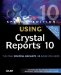# Using Brackets in Formulas

Regardless of which syntax is chosen, some fundamental concepts to formula creation are important.

Several variations of brackets are used within the formula language, and it can be confusing to know which one to use at a particular time. To clear up some of the confusion, here is a way to remember them phonetically:

• {} French = Fields

For example, {Table.Field} is used to refer to fields, formula fields or parameter fields in the report definition.

• [] Square = Selected

For example, {Table.Field} returns only the first character of a string field. Square brackets are used for indexes on array types (for example, strings or array data types).

• () Parenthesis = Parameters

For example, Function ({Table.Field}) passes the field to the function. Parentheses are used to define which parts of a calculation or formula should be performed first (that is, defines order of precedence for mathematical and non-mathematical operations).Special Edition Using Crystal Reports 10
ISBN: 0789731134
EAN: 2147483647
Year: 2003
Pages: 341